Experiment No: 04

Title: To design, build and test Schmitt trigger and plot transfer characteristic

AIM : To design, build and test RTD based bridge circuit and interface with instrumentation amplifier.

APPARATUS REQUIRED:

OPA544 Power Op-Amp, INA111 Instrumentation Amplifier, LF411 Op-amp, resistors, thermistor.

THEORY:

The differential amplifier isnâ€™t really very practical. The current that flows into the top input depends on the voltage applied to the bottom input. This may not seem that bad, but it is. It means that the input characteristics of this circuit are not constant. One way to get around this would be to place a voltage follower on each input, as shown here. Now this is a perfectly good circuit. If the two Rfs are closely matched and the two Rins are also closely matched, then this circuit will amplify differential voltages very well and reject Common voltages (a voltage that is common to both inputs should subtract out of the Equation. In EE terms, it has a good Common-Mode-Rejection-Ration (CMRR). But what if you want to change the gain? Youâ€™d have to change two resistors at the same time. By adding two more matched resistors and variable resistor weâ€™ll get the Instrumentation amplifier shown at right. The equation for this circuit is: This is an important circuit and you will probably see it again many times. For instance, if you had to amplify the output of a Wheatstone bridge of stain gages, this would be the amp for the job.

The figure shows a differential instrumentation amplifier using a transducer bridge. Resistance RT is a resistance of which changes proportional with some physical quantity Such as temperature, pressure, light intensity etc. RT is the resistance of the transducer &is the change in the resistance RT.

A DC or AC voltage source can excite the bridge. Under balanced conditions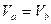------------------ (1)

But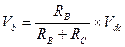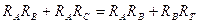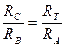------------------------ (2)

(Under balanced condition)

The values of RA, RB& RC are such that they are equal to RT at a particular value of the physical quantity being measured. This value is decided by the designer depending on the transducer characteristics.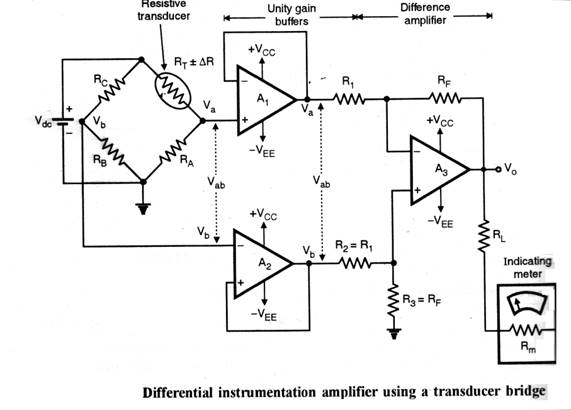###### PROCEDURE:
1. Design the circuit & connect it as shown in circuit diagram.
2. Vary the Temp & measure the voltage & respective resistance.
3. Note observation & plot the graph.

OBSERVATION TABLE:

 Sr.No. Temp Resistance Vo

Conclusion: